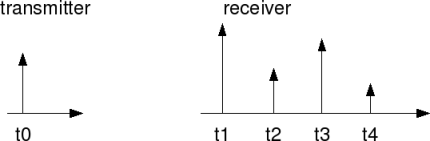- DSP log - http://www.dsplog.com -

Rayleigh multipath channel model

Posted By Krishna Sankar On July 14, 2008 @ 5:42 am In Channel | 196 Comments

The article gives a quick overview of a simple statistical multipath channel model called Rayleigh fading channel model.

## Multipath environment

In a multipath environment, it is reasonably intuitive to visualize that an impulse transmitted from transmitter will reach the receiver as a train of impulses.Figure: Impulse response of a multipath channel

Let the transmit bandpass signal be,
, where

is the baseband signal,
is the carrier frequency and
is the time.

As shown above, the transmit signal reaches the receiver through multiple paths where the path has an attenuation and delay . The received signal is,

.

Plugging in the equation for transmit baseband signal from the above equation,

.

The baseband equivalent of the received signal is,

,

where is the phase of the path.

The impulse response is,

.

The phase of each path can change by radian when the delay changes by . If is large, relative small motions in the medium can cause change of radians. Since the distance between the devices are much larger than the wavelength of the carrier frequency, it is reasonable to assume that the phase is uniformly distributed between 0 and radians and the phases of each path are independent (Sec 2.4.2 [WIRELESS-COMMUNICATION: TSE, VISWANATH]).

When there are large number of paths, applying Central Limit Theorem , each path can be modelled as circularly symmetric complex Gaussian random variable with time as the variable. This model is called Rayleigh fading channel model.

A circularly symmetric complex Gaussian random variable is of the form,

,

where real and imaginary parts are zero mean independent and identically distributed (iid) Gaussian random variables. For a circularly symmetric complex random variable ,

.

The statistics of a circularly symmetric complex Gaussian random variable is completely specified by the variance,

.

The magnitude which has a probability density,

is called a Rayleigh random variable.

This model, called Rayleigh fading channel model, is reasonable for an environment where there are large number of reflectors.

## Reference

[WIRELESS-COMMUNICATION: TSE, VISWANATH]Fundamentals of Wireless Communication, David Tse, Pramod ViswanathNote:
In a future post, we will try and derive the probability density function of sum of squares of independent Gaussian random variables